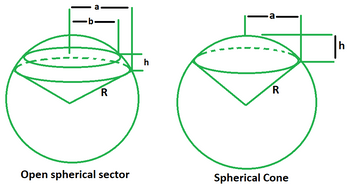Open in App
Not now

# Spherical Sector Formula

• Last Updated : 26 Apr, 2022

A spherical sector is a solid formed by rotating a sector of a circle along an axis that passes through the circle’s centre but has no points inside the sector. If the revolution axis is one of the radial sides, the resulting sector is a spherical cone; otherwise, it is an open spherical sector. A zone and one or two conical surfaces surround a spherical sector. A spherical sector is that part of the sphere that has a vertex at the centre and a conical boundary.### Spherical Sector Formulas

A spherical sector has two major formulae that are connected to its area and volume.

Total surface area formula (A)

The sum of the area of the zone and the lateral areas of the bounding cones is equal to the total surface area of a spherical sector.

A = Area of zone + Lateral area of bounding cones

A = 2πRh + πaR + πbR

A = πR (2h + a + b)

Now, for a spherical cone, b = 0. So, the area in that case is,

A = πR (2h + a)

Volume of a spherical sector formula

The volume of a spherical sector, whether open or conical, equals one-third of the product of the area of the zone and the radius of the sphere.

V = (1/3) × Area of zone × radius

V = (1/3) × 2πRh × R

V = 2πR2h/3

### Sample Problems

Problem 1. Find the total surface area of a sector of a sphere if its radius is 6 cm, the radius of the bounding cone is 8 cm and the height is 10 cm.

Solution:

We have, R = 6, h = 10 and a = 8.

Using the formula we have,

A = πR (2h + a)

= (22/7) (6) (20 + 8)

= (22/7) (6) (28)

= 528 sq. cm

Problem 2. Find the radius of the spherical sector if its total surface area is 2640 sq. cm, the radius of the bounding cone is 9 cm and the height is 7 cm.

Solution:

We have, V = 2640, h = 7 and a = 9.

Using the formula we have,

A = πR (2h + a)

=> 2640 = (22/7) (R) (14 + 9)

=> 2640 = (22/7) (R) (23)

=> 18480 = 506R

=> R = 18480/506

=> R = 36.52 cm

Problem 3. Find the volume of the spherical sector if its radius is 21 cm and height is 18 cm.

Solution:

We have, R = 21 and h = 18.

Using the formula we have,

V = 2πR2h/3

= 2 (22/7) (21) (21) (18) (1/3)

= 2 (22) (3) (21) (6)

= 16632 cu. cm

Problem 4. Find the height of the spherical sector if its volume is 660 cu. cm and radius is 12 cm.

Solution:

We have, V = 660 and R = 12.

Using the formula we have,

V = 2πR2h/3

=> 660 = 2 (22/7) (12) (12) (h) (1/3)

=> 660 = 2112h/7

=> 301.71h = 660

=> h = 2.18 cm

Problem 5. Find the volume of the spherical sector if its total surface area is 132 sq. cm, height is 7 cm and radius of the bounding cone is 4 cm.

Solution:

We have, A = 132, h = 7 and a = 4.

Using the formula we have,

A = πR (2h + a)

=> 132 = (22/7) (R) (18)

=> 396R = 924

=> R = 2.33

So, the volume is,

V = 2πR2h/3

= 2 (22/7) (2.33) (2.33) (7) (1/3)

= 238.87/3

= 79.62 cu. cm

My Personal Notes arrow_drop_up